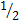# 3e551 Volume 4 Ure

98 Questions | Total Attempts: 83Settings• 1.
Using the laws of multiplication, what is the identity element in the equation 9 • 1 = 9?
• A.

1 = 1

• B.

0 • 1

• C.

1

• D.

0

• 2.
How many significant figures are in the exponential notation expression 3.200 x 10-5?
• A.

Two

• B.

Four

• C.

Five

• D.

Eight

• 3.
How many zeros are significant in the number 70.0700?
• A.

One

• B.

Two

• C.

Three

• D.

Four

• 4.
When removing a grouping symbol, preceded by a plus sign from an algebraic sum, what must you do to each term?
• A.

Make each term negative.

• B.

Make each term positive.

• C.

Nothing; each term sign stays the same.

• D.

Change each positive term to a negative, and each negative term to a positive.

• 5.
What is the reciprocal of  + ?
• A.

6/5

• B.

3/6+2/6

• C.

5/1

• D.

6/1

• 6.
Which of the following shows the factors of 3x2 – 6x
• A.

(2) (x2 – 3x)

• B.

(3x2) (x – 2)

• C.

(x) (3x – 2x)

• D.

(3x) (x – 2)

• 7.
What type of triangle has two of its sides equal in length?
• A.

Right

• B.

Scalene

• C.

Isosceles

• D.

Equilateral

• 8.
In a right angle, what type of angle is greater than 90o, but less than 180o?
• A.

Acute

• B.

Reflex

• C.

Obtuse

• D.

Straight

• 9.
What is the length of side a when b is equal to 6.5, and c is equal to 12.0?
• A.

10.09

• B.

11.25

• C.

42.25

• D.

101.75

• 10.
Convert 45 minutes to a decimal degree.
• A.

.25°

• B.

.45°

• C.

.75°

• D.

4.5°

• 11.
Solve the following right triangle for unknown angle.GIVEN:a 303.40’c 501.21’FIND: A
• A.

37 ° 15’ 11”

• B.

37 ° 25’ 30”

• C.

52 ° 44’ 49”

• D.

60 ° 53’ 35”

• 12.
If sides a, b, and c of an oblique triangle are known, which of the following functions would you use to solve for angle B
• A.

Law of Sines.

• B.

Law of Cosines.

• C.

Law of Tangents.

• D.

Pythagorean theorem.

• 13.
How many times, does the prefix “milli” refer to subdividing the basic unit in the metric system?
• A.

10

• B.

100

• C.

1,000

• D.

10,000

• 14.
Convert 12 square feet to its equivalent in square meters (m)?
• A.

1.11 m^2

• B.

10.03 m^2

• C.

14.35 m^2

• D.

129.17 m^2

• 15.
What are the two types of survey methods normally used?
• A.

Geodetic and Topographic.

• B.

Geodetic and Plane.

• C.

Geodetic and Triangulation.

• D.

Plane and Topographic.

• 16.
The control of survey networks for the United States are known as the
• A.

North American Datum of 1983 (NAD 83) for horizontal control points, and the North American Vertical Datum of 1988 (NAVD 88) for vertical control points.

• B.

North American Datum of 1987 (NAD 87) for horizontal control points, and the NAVD 88 for vertical control points.

• C.

NAD 83 for horizontal control points, and the North American Vertical Datum of 1989 (NAVD 89) for vertical control points.

• D.

NAD 83 for vertical control points, and the NAVD 88 for horizontal control points.

• 17.
The degree of accuracy for a horizontal measurement is determined from the
• A.

Measured value and its probable error.

• B.

Assumed value and its probable error.

• C.

Horizontal value and its assumed error.

• D.

Horizontal angular value and its assumed error.

• 18.
What type of error measures the difference between the measured and the true value of a quantity?
• A.

Residual

• B.

Resultant

• C.

Accidental

• D.

Systematic

• 19.
Which statement is true of surveying field notes?
• A.

They are never erased.

• B.

They are recorded in black ink if they are to be reproduced.

• C.

If they come from military personnel, they cannot be used as legal evidence.

• D.

They can be kept on scraps of paper and later transcribed to a permanent copy.

• 20.
How many feet long are most 100-foot steel tapes?
• A.

99

• B.

100

• C.

101

• D.

102

• 21.
What is the term used for measuring a 100-foot horizontal distance in a series of measurements that total 100 feet?
• A.

Short tape.

• B.

Partial tape.

• C.

Cutting tape.

• D.

Breaking tape.

• 22.
Which member of the taping party carries the tape forward to ensure that the tape is free of loops that could lead to kinks and breakage?
• A.

Recorder.

• B.

Rear tape person.

• C.

• D.

Instrument person.

• 23.
A tape is standardized at 99.98 feet. You measure between two established points, and thetape reads 100 feet. What would you do to apply the correction to the measurement?
• A.

• B.

Subtract

• C.

Divide

• D.

Multiply

• 24.
Use the formula below to find how many feet is in the actual distance between the points. The distance between two points was measured at 60.00 feet and the tape, supported only at the ends, weighs 2 pounds with 20 pounds per 100 feet of tension applied: C=
• A.

59.96

• B.

59.97

• C.

59.98

• D.

59.99

• 25.
Which ratio is not a stadia interval ratio?
• A.

1:100

• B.

1:200

• C.

1:300

• D.

1:333

Related TopicsBack to top